Question

In an experiment, one of the forces exerted on a proton is F⃗ =−αx2i^, where α=12N/m2. What is the potential-energy function for F⃗ ? Let U=0 when x=0.

1.The potential energy is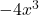Explanation:

Given that,

Force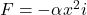We need to calculate the potential energy

Using formula of work done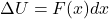Put the value of F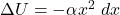On integration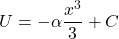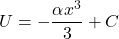…(I)

U = 0, x = 0 so C = 0

Put the value of c and α in equation (I)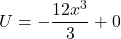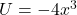Hence, The potential energy is